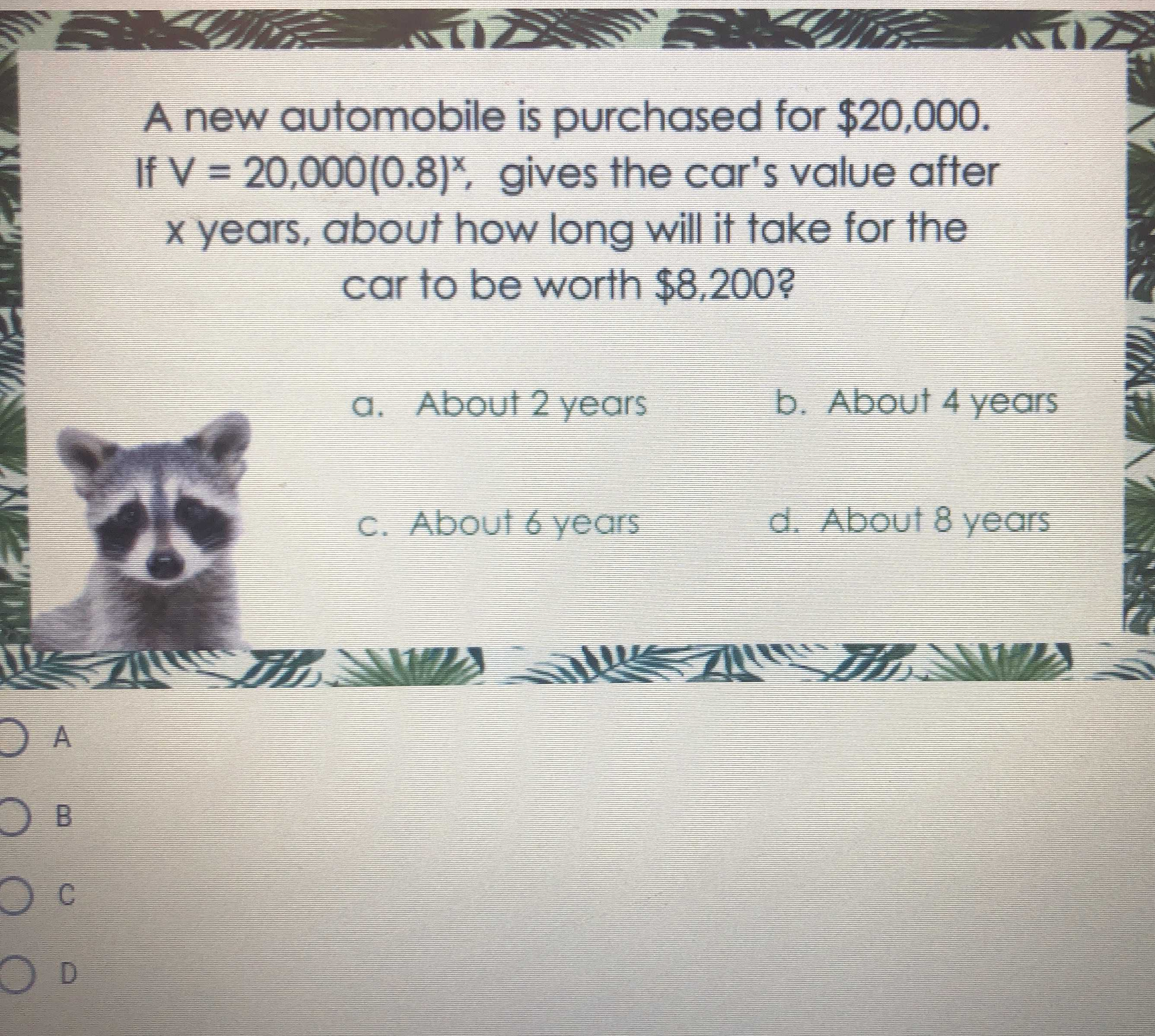### ¿Todavía tienes preguntas de matemáticas?

Pregunte a nuestros tutores expertos
Algebra
PreguntaA new automobile is purchased for $$\ 20,000$$ . If $$V = 20,000 ( 0.8 ) ^ { x }$$ , gives the car's value after $$x$$ years, about how long will it take for the car to be worth $$\ 8,200$$ ?

a. About $$2$$ years b. About $$4$$ years c. About $$6$$ years d. About $$8$$ years